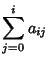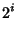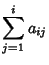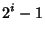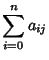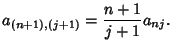## Figurate Number Triangle

A Pascal's Triangle written in a square grid and padded with zeroes, as written by Jakob Bernoulli(Smith 1984). The figurate number triangle therefore has entries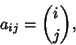where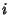is the row number,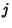the column number, and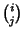a Binomial Coefficient. Written out explicitly (beginning each row with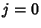),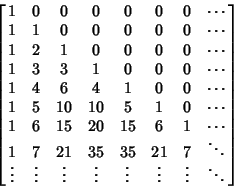Then we have the sum identities